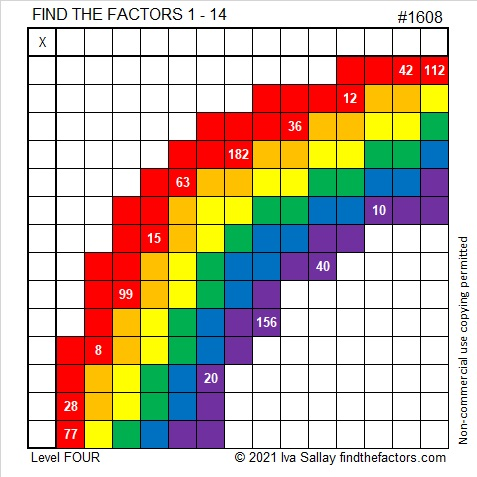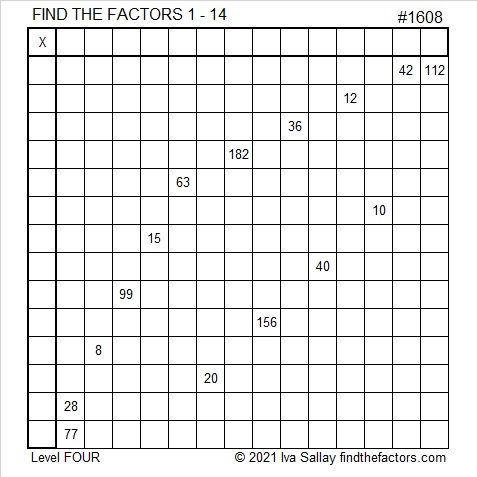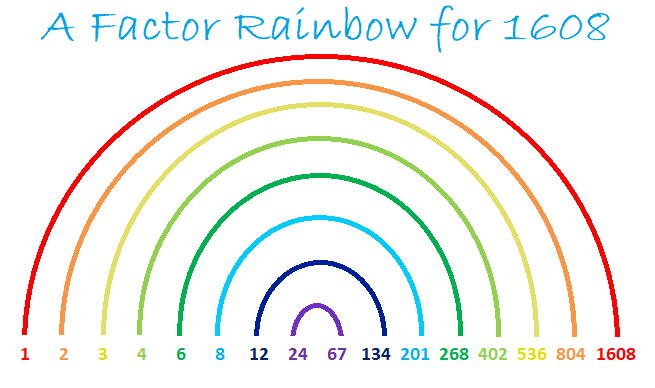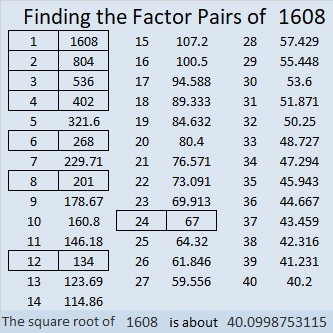# 1608 Rainbow

Contents

### Today’s Puzzle:

We often think of rainbows around Saint Patrick’s Day. Here is a rainbow puzzle for you to solve. It won’t be all that easy even if I tell you that
13 × 14 = 182,
12 × 13 = 156, and
8 ×  14 = 112.

Good luck!If you’d like to print the puzzle but not use so much ink, here’s a puzzle with all the same clues:Print the puzzles or type the solution in this excel file: 14 Factors 1604-1612.

### Factor Rainbow for 1608:

The number 1608 has enough factors to make an impressive factor rainbow:### Factors of 1608:

• 1608 is a composite number.
• Prime factorization: 1608 = 2 × 2 × 2 × 3 × 67, which can be written 1608 = 2³ × 3 × 67.
• 1608 has at least one exponent greater than 1 in its prime factorization so √1608 can be simplified. Taking the factor pair from the factor pair table below with the largest square number factor, we get √1608 = (√4)(√402) = 2√402.
• The exponents in the prime factorization are 3,1 and 1. Adding one to each exponent and multiplying we get (3 + 1)(1 + 1) (1 + 1) = 4 × 2 × 2 = 16. Therefore 1608 has exactly 16 factors.
• The factors of 1608 are outlined with their factor pair partners in the graphic below.### More about the Number 1608:

1608 is the difference of two squares in four different ways:
403² – 401² = 1608,
203² – 199² = 1608,
137² – 131² = 1608, and
73² – 61² = 1608.

This site uses Akismet to reduce spam. Learn how your comment data is processed.